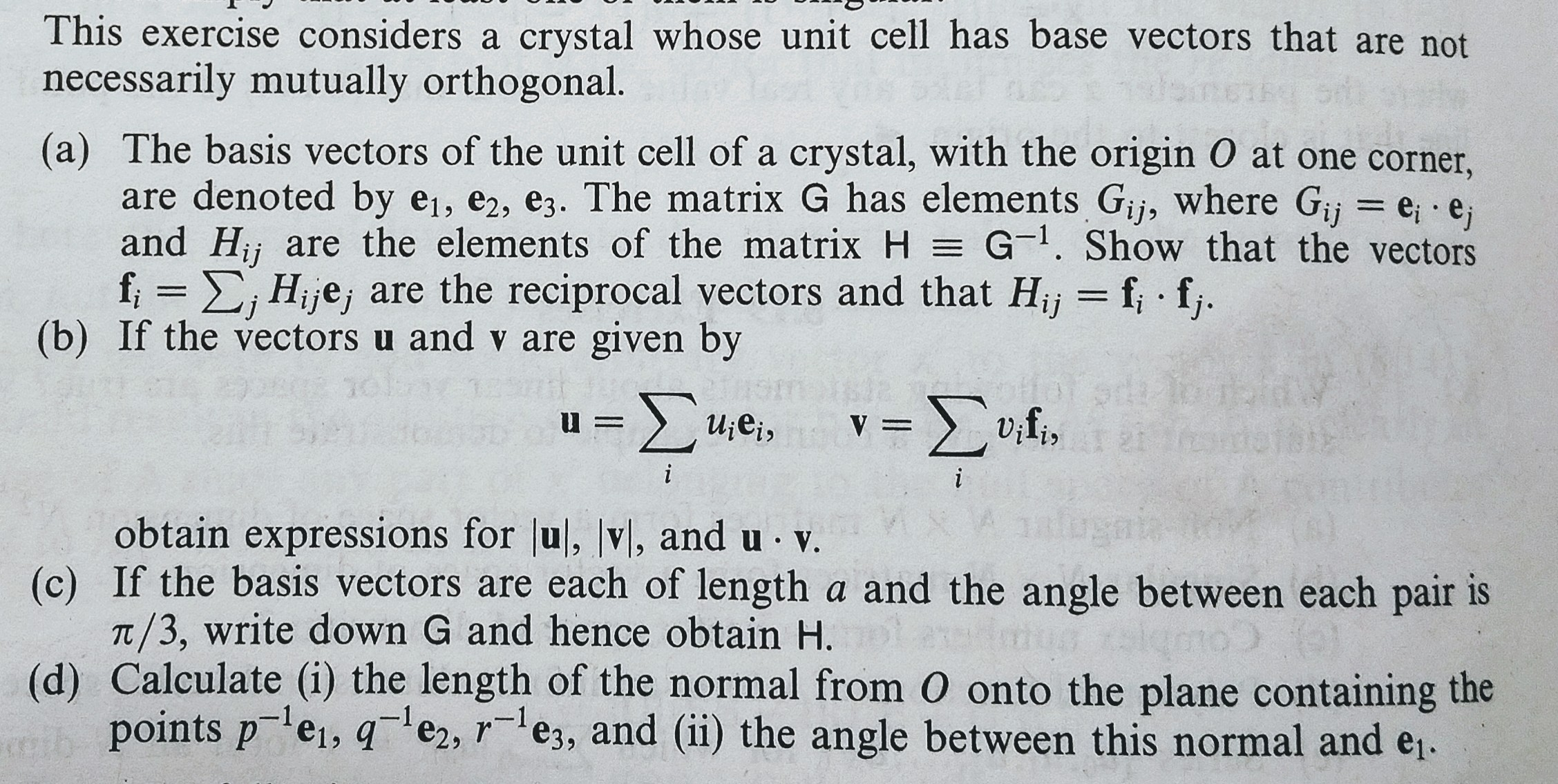This exercise considers a crystal whose unit cell has base vectors that are notnecessarily mutually orthogonal(a) The basis vectors of the unit cell of a crystal, with the origin O at one corner,are denoted by ei, e2 , ез. The matrix G has elements Gijs where Gij ei-ejand Hj are the elements of the matrix HG1. Show that the vectorsf , Hije, are the reciprocal vectors and that Hj f f(b) If the vectors u and v are given by01-1obtain expressions for lul, vl, and u v.T/3, write down G and hence obtain H.points p ei, qe2, es, and (ii) the angle between this normal and e,.(c) If the basis vectors are each of length a and the angle between each pair is(d) Calculate (i) the length of the normal from O onto the plane containing the

Questionhelp_outlineImage TranscriptioncloseThis exercise considers a crystal whose unit cell has base vectors that are not necessarily mutually orthogonal (a) The basis vectors of the unit cell of a crystal, with the origin O at one corner, are denoted by ei, e2 , ез. The matrix G has elements Gijs where Gij ei-ej and Hj are the elements of the matrix HG1. Show that the vectors f , Hije, are the reciprocal vectors and that Hj f f (b) If the vectors u and v are given by 0 1-1 obtain expressions for lul, vl, and u v. T/3, write down G and hence obtain H. points p ei, qe2, es, and (ii) the angle between this normal and e,. (c) If the basis vectors are each of length a and the angle between each pair is (d) Calculate (i) the length of the normal from O onto the plane containing the fullscreen
Step 1

To discuss the properties of the reciprocal basis to the given basis of vectors

Step 2

We are given a system of basis vectors e(1),e(2),e(3) and the matrices G and H are defined as above.

Step 3

By definition, the reciprocal system of vectors f1, f2,f3 are characterized b...

Want to see the full answer?

See Solution

Want to see this answer and more?

Our solutions are written by experts, many with advanced degrees, and available 24/7

See Solution
Tagged in

Math